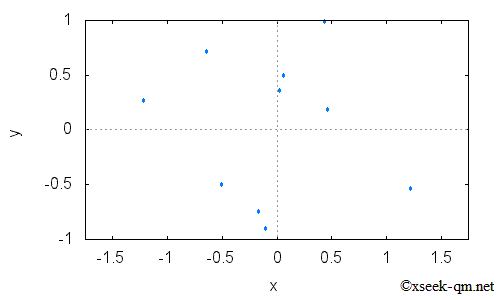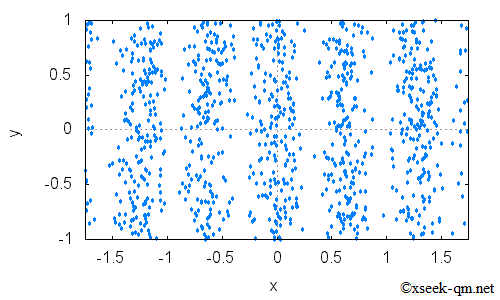# The double slit experiment of quantum mechanics

2014/1/26
Published 2002/2/14

I would like to guide you to the entrance of the quantum theory in this site. I would like to write the topics for the people who are not familiar with physics. I hope you will be interested in quantum theory.

## The double slit experiment

At first, I would like to introduce the double slit experiment of quantum mechanics.An electron flies against a wall in the above figure. The wall has two slits. The electron hits the wall or passes through the slits. The electron that passed through the slits hits another wall. What happens if many electrons pass through the slits? Some electrons hit the wall as shown in the following figure.If we increase the number of electrons hitting the wall, the above figure changes into the following figure.There is a stripe pattern. We can see the distribution of electrons in the following figure.What made this stripe pattern? In fact, electrons have a nature of wave. Waves that passed through two slits construct or destruct. We call the phenomena interference. The interference of the wave made the stripe pattern. This experiment is called "the double slit experiment". I think that this result is very mysterious. The electrons pass through the slit, one by one. Why can we see the stripe pattern caused by interference?

## Why is the stripe pattern caused by interference made?

The wave of the electron is expressed by the following function.

Ψ (x)

This function is This function is called wave function and pronounced as "psi of x". The variable x is a real number which describe the position of the electron. The wave function is the function of complex numbers. The wave function describe a state of the electron.

In the double slit experiment, the electron has two passes. The first pass is a pass through the right slit. The second pass is the other pass through the left slit. We describe the wave function of the wave through the right slit as ΨR (x ). We describe the wave function of the wave through the left slit as ΨL (x ). We add these two wave functions ΨR (x) and ΨL (x).

Ψ (x) = ΨR (x) + ΨL (x)

The interference occurs from this summation. It is called "superposition principle." Observation probability of electrons, P(x) is the square of the absolute value of the wave function.

P (x ) = |Ψ (x ) |2

Why is the observation probability of electrons the square of the absolute value of the wave function?

Physicist Feynman described the following words in 1965 in the textbook "The Feynman Lectures on Physics".

One might still like to ask: "How does it work? What is the machinery behind the law?"

No one has found any machinery behind the law. No one can "explain" any more than we have just "explained." No one will give you any deeper representation of the situation. We have no ideas about a more basic mechanism from which these results can be deduced.

So, we do not know the mechanism. I would like to quote Einstein's words about 1951 from the textbook "Gauge Theories in Particle Physics".

All the fifty years of conscious brooding have brought me no closer to the answer to the question, "What are light quanta?" Of course today every rascal thinks he knows the answer, but he is deluding himself.

In this site, I would like to consider the mystery of the quantum theory with you.

Related Articles: